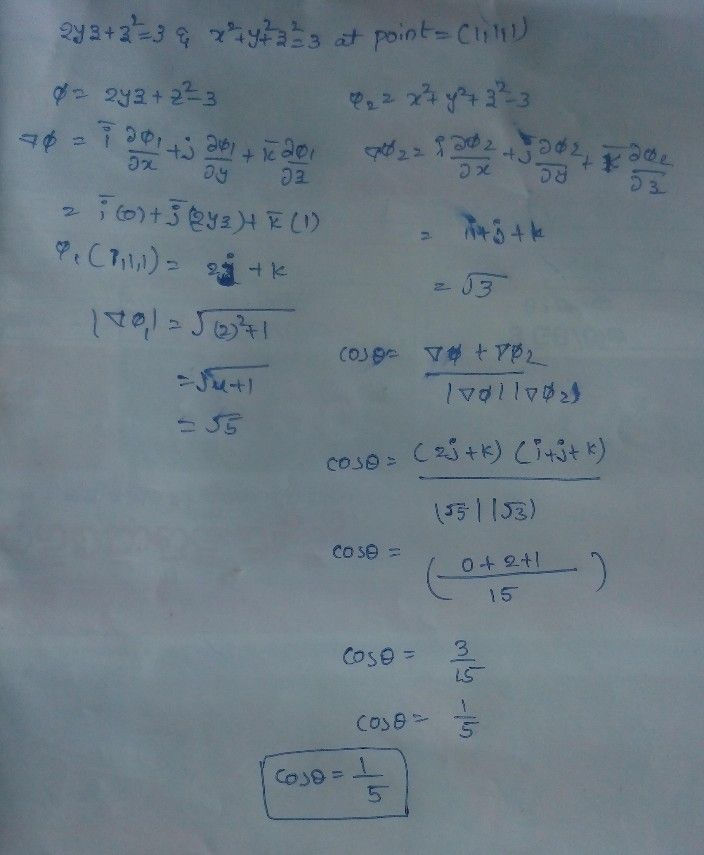Symbol
Problem$∠$ Not yet answered Marked out of 10.0 P Flag gaestion Determine the angle between the surfaces $2yz+z^{2}=3$ and $x^{2}+y^{2}+z^{2}=3$ at the point(1, $\left(1$ $1,$ $1\right)$
Calculus
SolutionQanda teacher - bhargavi